# 1637 Flower Challenge

### Today’s Puzzle:

Mother’s Day in the United States is this Sunday so I made this challenging flower puzzle for the occasion.

Use logic to write the numbers from 1 to 10 in each of the four boldly outlined areas so that those numbers and the given clues work together to make four multiplication tables.### Factors of 1637:

• 1637 is a prime number.
• Prime factorization: 1637 is prime.
• 1637 has no exponents greater than 1 in its prime factorization, so √1637 cannot be simplified.
• The exponent in the prime factorization is 1. Adding one to that exponent we get (1 + 1) = 2. Therefore 1637 has exactly 2 factors.
• The factors of 1637 are outlined with their factor pair partners in the graphic below.

How do we know that 1637 is a prime number? If 1637 were not a prime number, then it would be divisible by at least one prime number less than or equal to √1637. Since 1637 cannot be divided evenly by 2, 3, 5, 7, 11, 13, 17, 19, 23, 29, 31, or 37, we know that 1637 is a prime number.### More about the Number 1637:

1637 is the sum of two squares:
31² + 26² = 1637.

1637 is the hypotenuse of a Pythagorean triple:
285-1612-1637, calculated from 31² – 26², 2(31)(26), 31² + 26².

Here’s another way we know that 1637 is a prime number: Since its last two digits divided by 4 leave a remainder of 1, and 31² + 26² = 1637 with 31 and 26 having no common prime factors, 1637 will be prime unless it is divisible by a prime number Pythagorean triple hypotenuse less than or equal to √1637. Since 1637 is not divisible by 5, 13, 17, 29, or 37, we know that 1637 is a prime number.

Do you notice anything else special about the number 1637 in this color-coded chart?# 1623 Easter Basket Challenge Puzzle

### Today’s Puzzle:

Since I’ve recently made puzzles with a pink, purple, or blue Easter egg as well as some blades of grass blowing in the spring wind, it only makes sense that I would also give you an Easter basket in which to hold those other puzzles.

The puzzle is solved if you have written the numbers 1 to 10 in each of the boldly outlined areas of the puzzle, and if those numbers work with the clues to form four multiplication tables.Print the puzzles or type the solution in this excel file: 12 Factors 1614-1623.

If you need a little help, here’s the same puzzle with the factor pairs for the clues written in.And if you want even more help, here’s a 2 1/2 minute video on how to get started. I assume you already know the directions on how to solve this kind of puzzle that I gave at the top of this post.

### Factors of 1623:

• 1623 is a composite number.
• Prime factorization: 1623 = 3 × 541.
• 1623 has no exponents greater than 1 in its prime factorization, so √1623 cannot be simplified.
• The exponents in the prime factorization are 1 and 1. Adding one to each exponent and multiplying we get (1 + 1)(1 + 1) = 2 × 2 = 4. Therefore 1623 has exactly 4 factors.
• The factors of 1623 are outlined with their factor pair partners in the graphic below.

### More About the Number 1623:

1623 is the hypotenuse of a Pythagorean triple:
1023-1260-1623, which is 3 times (341-420-541).

# 1603 Challenge Puzzle

### Today’s Puzzle:

Use logic to write the numbers 1 to 10 in each of the four boldly outlined areas so that the puzzle functions like four multiplication tables that work together.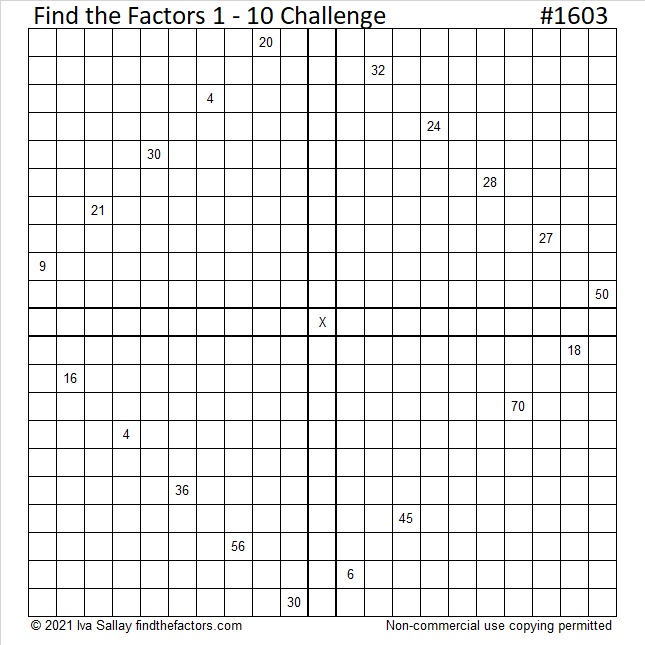Print the puzzles or type the solution in this excel file: 10 Factors 1594-1603.

### Factors of 1603:

• 1603 is a composite number.
• Prime factorization: 1603 = 7 × 229.
• 1603 has no exponents greater than 1 in its prime factorization, so √1603 cannot be simplified.
• The exponents in the prime factorization are 1 and 1. Adding one to each exponent and multiplying we get (1 + 1)(1 + 1) = 2 × 2 = 4. Therefore 1603 has exactly 4 factors.
• The factors of 1603 are outlined with their factor pair partners in the graphic below.### More about the Number 1603:

1603 is the hypotenuse of a Pythagorean triple:
420-1547-1603, which is 7 times (60-221-229).

# 1584 You Will L♥ve This Challenge Puzzle!

### Today’s Puzzle:

Here’s a Valentine’s challenge for you: Can you write the numbers from 1 to 10 in each of the four areas of the puzzle that touch the box with the X so that the numbers you write make the puzzle become four multiplication tables? It won’t be easy, but remember to use logic. If you succeed, you will absolutely lve the puzzle!### 1584 Factor Cake:

1584 obviously is divisible by 2. It is divisible by 4 because 84 is divisible by 4.
It is divisible by 8 because 84 is not divisible by 8 and 5 is odd.
1584 is divisible by 3 and by 9 because 1 + 5 + 8 + 4 = 18, a number divisible by 3 and by 9.
1584 is divisible by 11 because 1 – 5 + 8 – 4 = 0.

Thus, 1584 makes a very tall and very nice factor cake with a couple of candles on top!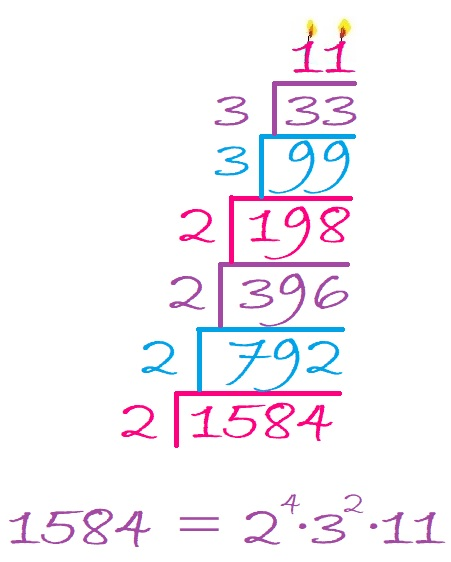### Factors of 1584:

• 1584 is a composite number.
• Prime factorization: 1584 = 2 × 2 × 2 × 2 × 3 × 3 × 11, which can be written 1584 = 2⁴ × 3² × 11.
• 1584 has at least one exponent greater than 1 in its prime factorization so √1584 can be simplified. Taking the factor pair from the factor pair table below with the largest square number factor, we get √1584 = (√144)(√11) = 12√11.
• The exponents in the prime factorization are 4, 2 and 1. Adding one to each exponent and multiplying we get (4 + 1)(2 + 1)(1 + 1) = 5 × 3 × 2 = 30. Therefore 1584 has exactly 30 factors.
• The factors of 1584 are outlined with their factor pair partners in the graphic below.### More about the Number 1584:

1584 is the difference of two squares NINE different ways:
397² – 395² = 1584,
200² – 196² = 1584,
135² – 129² = 1584,
103² – 95² = 1584,
72² – 60² = 1584,
53² – 35² = 1584,
47² – 25² = 1584,
45² – 21² = 1584, and
40² – 4² = 1584.
Yes, we are only 16 numbers away from 1600, the next perfect square!

1584 is in this cool pattern: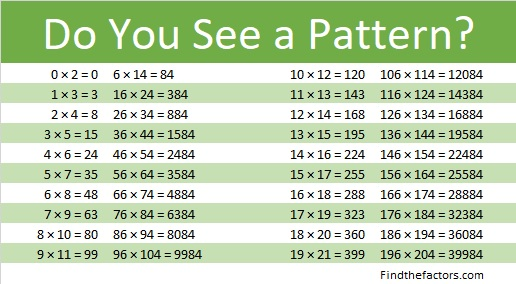# 1568 A Challenging Christmas Tree

### Today’s Puzzle:

Can you write the numbers from 1 to 10 in each of the boldly outlined columns and rows so that each quadrant of this Christmas tree puzzle behaves like a multiplication table? Remember to use logic and not guessing and checking.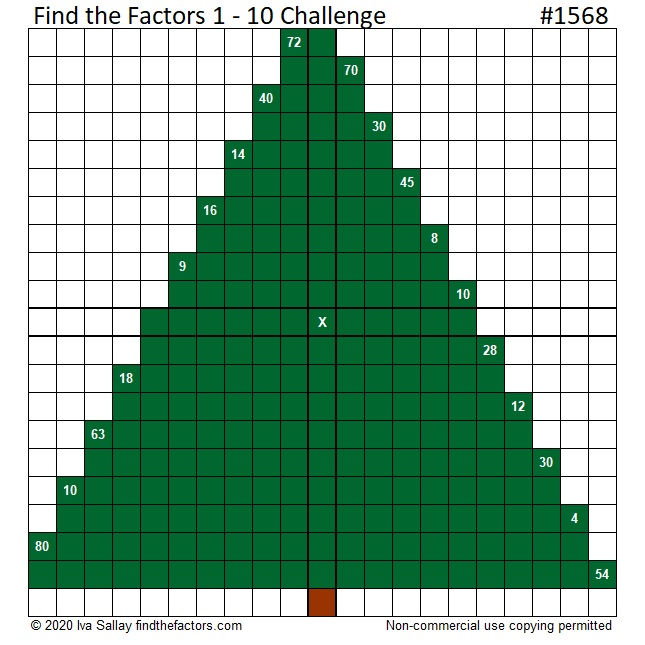Here is the same puzzle that won’t use so much ink to print:### 1568 Factor Tree:

Here is one of several possible factor trees for 1568: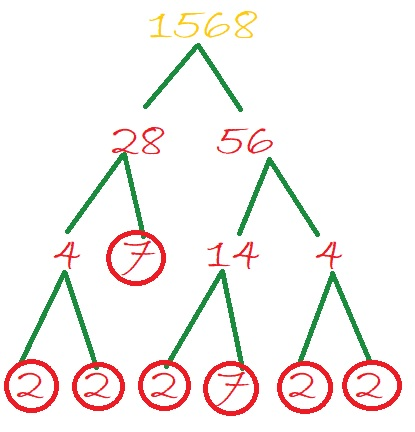### Factors of 1568:

• 1568 is a composite number.
• Prime factorization: 1568 = 2 × 2 × 2 × 2 × 2 × 7 × 7, which can be written 1568 = 2⁵ × 7².
• 1568 has at least one exponent greater than 1 in its prime factorization so √1568 can be simplified. Taking the factor pair from the factor pair table below with the largest square number factor, we get √1568 = (√784)(√2) = 28√2.
• The exponents in the prime factorization are 5 and 2. Adding one to each exponent and multiplying we get (5 + 1)(2 + 1) = 6 × 3 = 18. Therefore 1568 has exactly 18 factors.
• The factors of 1568 are outlined with their factor pair partners in the graphic below.### More about the Number 1568:

1568 is the difference of two squares SIX different ways:
393² – 391² = 1568,
198² – 194² = 1568,
102² – 94² = 1568,
63² – 49² = 1568,
57² – 41² = 1568, and
42² – 14² = 1568.

# 1535 Your Favorite Candy Bar

### Today’s Puzzle:

If you could have your favorite candy bar in the largest size possible, would it actually turn out to be more than you can chew? Of course not. Even if it took a long time for you to finish that candy bar, it would not be an impossible challenge.

Think of this Challenge puzzle the same way. I think this one uses some delightful logic. Find that logic, take your time, and enjoy the process!Print the puzzles or type the solution in this excel file: 10 Factors 1526-1535

### Factors of 1535:

• 1535 is a composite number.
• Prime factorization: 1535 = 5 × 307.
• 1535 has no exponents greater than 1 in its prime factorization, so √1535 cannot be simplified.
• The exponents in the prime factorization are 1 and 1. Adding one to each exponent and multiplying we get (1 + 1)(1 + 1) = 2 × 2 = 4. Therefore 1535 has exactly 4 factors.
• The factors of 1535 are outlined with their factor pair partners in the graphic below.### Another Fact about the number 1535:

1535 is the hypotenuse of a Pythagorean triple:
921-1228-1535 which is (3-4-5) times 307.

# 1510 Challenge Puzzle

### Today’s Puzzle:

Challenge puzzles are like four multiplication tables connected to each other. Use logic to place the factors 1 to 10 in each boldly outlined column or row so that the given clues are the products of the factors you write. I hope you enjoy solving this puzzle as much as I enjoyed making it for you!Here’s an excel file with this week’s puzzles: 10 Factors 1502-1510

### Factors of 1510:

• 1510 is a composite number.
• Prime factorization: 1510 = 2 × 5 × 151.
• 1510 has no exponents greater than 1 in its prime factorization, so √1510 cannot be simplified.
• The exponents in the prime factorization are 1, 1, and 1. Adding one to each exponent and multiplying we get (1 + 1)(1 + 1)(1 + 1) = 2 × 2 × 2 = 8. Therefore 1510 has exactly 8 factors.
• The factors of 1510 are outlined with their factor pair partners in the graphic below.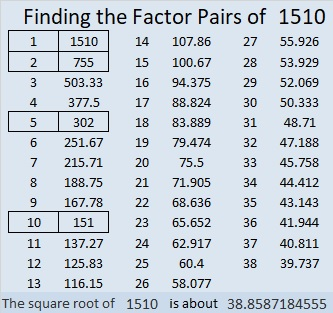### One More Fact about the Number 1510:

1510 is the hypotenuse of a Pythagorean triple:
906-1208-1510, which is (3-4-5) times 302.

# 1499 Challenge Puzzle

### Today’s Puzzle:

Use the 19 clues, logic, and the multiplication facts from a 10 × 10 multiplication table to find the unique solution of this Find the Factors Challenge puzzle. Good luck!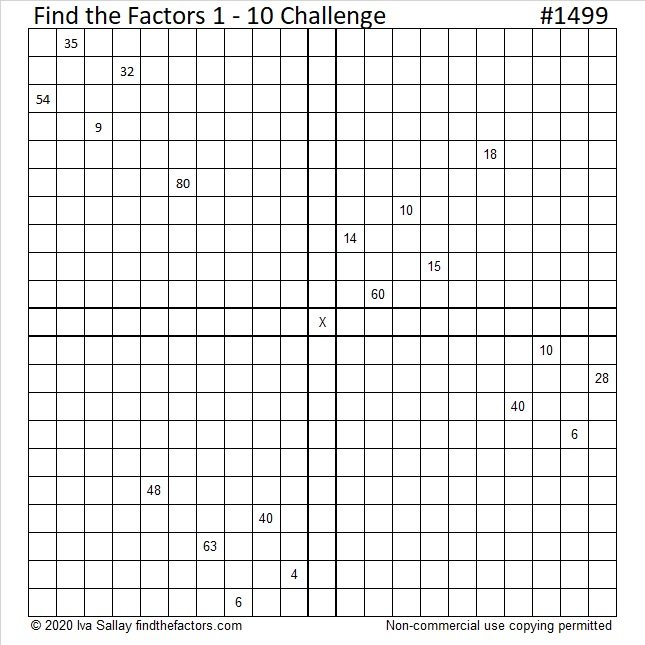### Factors of 1499:

• 1499 is a prime number.
• Prime factorization: 1499 is prime.
• 1499 has no exponents greater than 1 in its prime factorization, so √1499 cannot be simplified.
• The exponent in the prime factorization is 1. Adding one to that exponent we get (1 + 1) = 2. Therefore 1499 has exactly 2 factors.
• The factors of 1499 are outlined with their factor pair partners in the graphic below.How do we know that 1499 is a prime number? If 1499 were not a prime number, then it would be divisible by at least one prime number less than or equal to √1499. Since 1499 cannot be divided evenly by 2, 3, 5, 7, 11, 13, 17, 19, 23, 29, 31, or 37, we know that 1499 is a prime number.

### More Facts about the Number 1499:

1499 is the difference of two consecutive squares:
750² – 749² = 1499

oeis.org reminds us that 149, 199, and 499 are also prime numbers, so taking away one digit from 1499 always leaves a prime number.

# 1487 A Challenging Puzzle

### Today’s Puzzle:

This puzzle is like four out-of-order 1 – 10 multiplication tables that work together. The clues in the puzzle need to be the products of the numbers you write. It won’t be easy, but use logic to solve it. Good luck!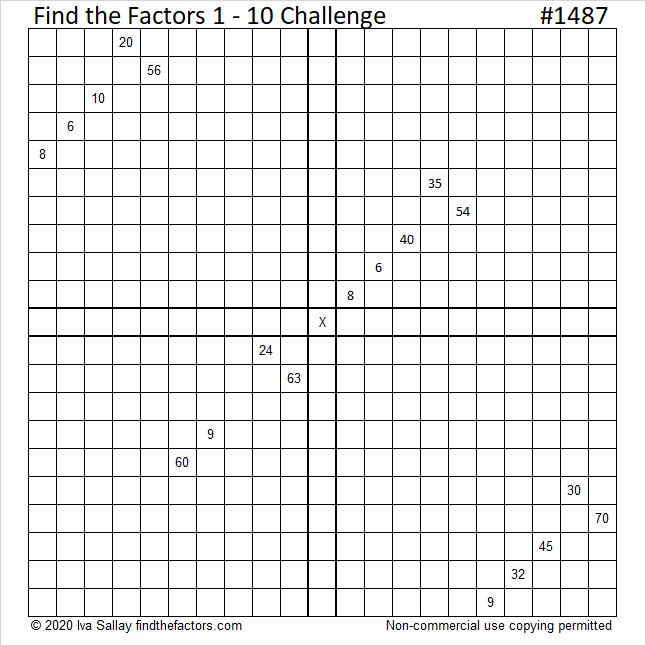Print the puzzles or type the solution in this excel file: 10 Factors 1478-1487

### Factors of 1487:

• 1487 is a prime number.
• Prime factorization: 1487 is prime.
• 1487 has no exponents greater than 1 in its prime factorization, so √1487 cannot be simplified.
• The exponent in the prime factorization is 1. Adding one to that exponent we get (1 + 1) = 2. Therefore 1487 has exactly 2 factors.
• The factors of 1487 are outlined with their factor pair partners in the graphic below.How do we know that 1487 is a prime number? If 1487 were not a prime number, then it would be divisible by at least one prime number less than or equal to √1487. Since 1487 cannot be divided evenly by 2, 3, 5, 7, 11, 13, 17, 19, 23, 29, 31, or 37, we know that 1487 is a prime number.

### Other Facts about the Number 1487:

1487 is the difference of two squares:
744²-743² = 1487.

1487 is the third prime number in the fifth prime decade. See it in the list below: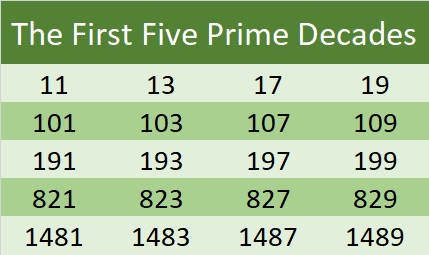# 1477 Challenge

### Today’s Puzzle:

It’s been a few days since I’ve last looked at this puzzle, but I was still able to solve it in 17 minutes. Can you beat my time?Print the puzzles or type the solution in this excel file: 12 Factors 1468-1477

### Factors of 1477:

• 1477 is a composite number.
• Prime factorization: 1477 = 7 × 211
• 1477 has no exponents greater than 1 in its prime factorization, so √1477 cannot be simplified.
• The exponents in the prime factorization are 1, and 1. Adding one to each exponent and multiplying we get (1 + 1)(1 + 1) = 2 × 2 = 4. Therefore 1477 has exactly 4 factors.
• The factors of 1477 are outlined with their factor pair partners in the graphic below.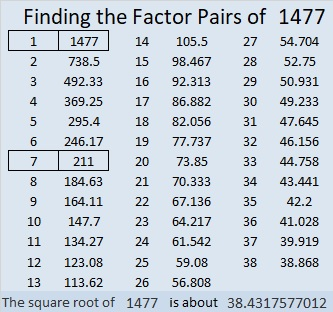### Another Fact about the Number 1477:

1477 is the difference of two squares in two different ways:
739² – 738² = 1477
109² – 102² = 1477